# 通卡在线(补充)

• 通过完成必修课或预备课，在秋季赶上或获得更大的灵活性
• 通过参加预科班或沉浸在具有挑战性的AP课程中，实现跨越式发展。
• 追求与你的激情一致的选修课，或探索一个全新的主题
• 灵活地获得你的体育或其他必要的课程学分，这样你就可以在你的日程安排中安排你想要的一切。
• 确保在大三和大四期间有时间参加签名活动

## 课程列表

*选择学期 S=夏季, F=秋季*选择学期S=夏季，F=秋季

*选择学期S=夏季，W=冬季
*在线，在第二部分之前完成第一部分。

*选择学期S=夏季，F=秋季

*选择学期S=夏季，W=冬季
*在线，在第二部分之前完成第一部分。*在线，在第二部分之前完成第一部分。

AP艺术史允许学生研究与不同时期的各种文化有关的主要艺术表现形式。*选择学期 F=秋季

*选择学期W=冬季
*在线，在第二部分之前完成第一部分。*在线，在第二部分之前完成第一部分。*选择学期 F=秋季，W=冬季*选择学期S=夏季，F=秋季，W=冬季

*选择学期S=夏季，F=秋季，W=冬季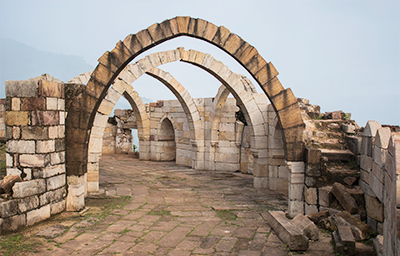*选择学期S=夏季，F=秋季

*选择学期S=夏季，W=冬季*选择学期 S=夏季, F=秋季, W=冬季*选择学期S=夏季，F=秋季

*选择学期S=夏季，W=冬季
*在线，在第二部分之前完成第一部分。*在线，在第二部分之前完成第一部分。*选择学期S=夏季，F=秋季，W=冬季

*选择学期S=夏季，F=秋季，W=冬季
*在线，在第二部分之前完成第一部分。

9-12#4014, S2

*选择学期 S=夏季*, F=秋季, W=冬季

*选择学期S=夏季*，F=秋季，W=冬季
*联系您所在大楼的高级学习人员，了解暑期数学课程的注册程序。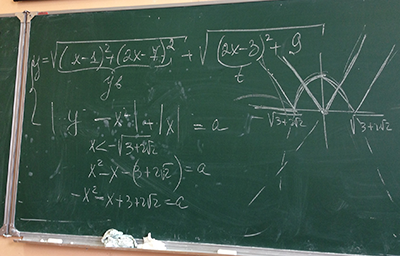*选择学期S=夏季，F=秋季，W=冬季

*选择学期S=夏季，F=秋季，W=冬季
*在线，在第二部分之前完成第一部分。

9年级, 10年级, 11年级, 12年级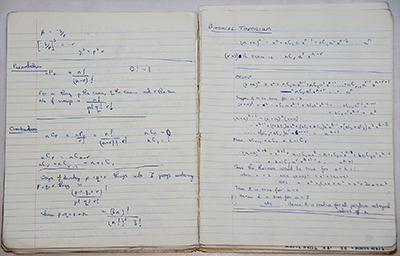*选择学期S=夏季，F=秋季，W=冬季

*选择学期S=夏季，F=秋季，W=冬季
*在线，在第二部分之前完成第一部分。

9年级, 10年级, 11年级, 12年级*选择学期S=夏季，F=秋季

*选择学期S=夏季，W=冬季*选择学期 F=秋季, W=冬季, , S=夏季

*选择学期F=秋天, W=冬天, , S=夏天
*在线，在第二部分之前完成第一部分。*选择学期S=夏季，F=秋季，W=冬季

*选择学期S=夏季，F=秋季，W=冬季
*在线，在第二部分之前完成第一部分。

9年级, 10年级, 11年级, 12年级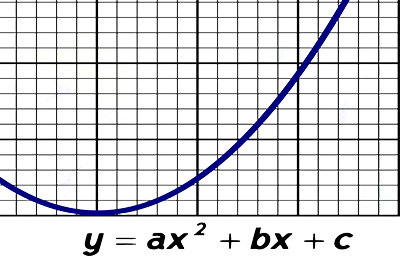*选择学期S=夏季，F=秋季，W=冬季

*选择学期S=夏季，F=秋季，W=冬季
*在线，在第二部分之前完成第一部分。

9-12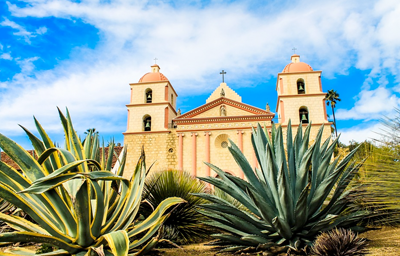*在线，在第二部分之前完成第一部分

*选择术语F=秋季，W=冬季### 联系信息

ben.stanerson@minnetonkaschools.org
952-237-9347

## 学术标准

Minnetonka公立学校遵守由明尼苏达州教育部（MDE）制定的学术标准。